## A SAS Macro to Perform Bootstrapping for Internal Validation of Time-Dependent Area Under the Curve

Started ‎03-15-2021 by
Modified ‎12-10-2021 by
Views 1,595
Paper 1019-2021
Authors
Jialin Han, Stanford University; Jiali Ling, University of Arizona; Maria E. Montez-Rath, Stanford University; Margaret R. Stedman, Stanford University

# ABSTRACT

We sought to develop an algorithm in SAS that uses the bootstrap for validation of a time-to-event prediction model and, in particular, to measure discrimination, which is the model’s ability to distinguish between those who develop and those who do not develop the outcome of interest at a specific time t. The area under the curve at time t, AUC(t), is a statistic typically used to estimate discrimination, that can be implemented in SAS using the ROCOPTIONS command in PHREG. However, the AUC(t) is computed directly from the data used to develop the model so that overfitting is common. Overfitting occurs when the model is excessively complex, e.g., by including too many predictors and as a consequence, the model performs poorly when applied to other data. To assess the amount of overfitting, one can perform internal validation, using the same dataset, or external validation, using a new dataset. Here, we present a SAS Macro to compute a validated AUC(t) using the bootstrap method. The bias corrected AUC(t) is calculated by applying the Cox Proportional Hazards Model to the bootstrapped data and applying the parameter estimates to the original data. The macro generates an estimate of the bias, the bootstrapped distribution of the AUC(t) and displays the original AUC(t) and the bias-corrected AUC(t) in an output table.

# INTRODUCTION

It is important to evaluate the performance of a prediction model. For a model with a binary outcome, the most commonly used statistic to measure discrimination is the concordance statistics (C-statistic). The C-statistic takes all possible pairs of individuals, one with the outcome of interest and the other without and calculates the proportion of pairs whose observed and predicted outcomes agree (higher predicted risk for those with the outcome). The higher the C-statistic, the better the model discriminates individuals who experience the outcome of interest from those who do not. The receiver operator characteristic (ROC) curve also measures discrimination of the predication model, by plotting the sensitivity versus 1 minus the specificity.  The sensitivity is the proportion of individuals who were predicted to be positive for the outcome out of the total individuals who are observed to be positive.  The specificity is the proportion of individuals who predicted as negative for the outcome out of the total individuals who are observed to be negative. Area under the curve (AUC), which equals the C-statistic, is computed by estimating the area under the ROC curve, essentially summarizing the entire curve into a single statistic.

In many epidemiological studies the outcome of interest is time to event, such as time to tumor detection or time to transplant. Since model accuracy could vary over the follow-up period and ROC curves are computed at specific time points, several approaches for estimating time-dependent ROC curves have been proposed, for example, the Kaplan Meier (KM) estimator, then Nearest Neighbor Estimator and the Inverse probability of Censoring Weighting estimator (Heagerty, 2000; Uno, 2007). These approaches are supported in SAS PHREG with ROCOPTIONS (Guo, 2017). In this paper, we estimate the time-dependent sensitivity and specificity using the KM estimator.

Validation is an important part in the model development process because it checks the accuracy of the model’s performance. This can be done either using external data, where new data is available, or with internal data, using the original sample. In practice, external validation is hard to implement since it requires extra time and data collection, so, internal validation is recommended to be done at the time of model development. There are several techniques for internal validation: split-sample, cross-validation, bootstrapping. Some research has shown that bootstrapping is recommended for estimation of the ROC curve by internal validation because it provides stable estimates with low bias (Steyerberg, 2001). In this paper, we developed a SAS Macro to perform internal validation of the AUC(t) by the bootstrap method.

# TIME DEPENDENT ROC CURVE

In SAS, we can use the PROC PHREG procedure with the PLOTS=ROC option to obtain time-dependent ROC curves. The ROCOPTIONS option offers various control mechanisms to specify the time points for ROC curves (AT=), the method of calculating ROC curves (Method=), and the AUC(t) statistics (AUC). In this paper, we selected the Kaplan-Meier method for both estimation and validation of the time dependent ROC curve.

The following statements can be used to run a Cox model and request the calculation of the AUC. Below, time2event is the time to the first event. Event is a binary indicator for having the event (1 = yes, 0 = no). Age and sex are the predictors in the model. As output, it provides the AUC at 365 days. Additionally, there is an option for SAS to output sensitivity and specificity by adding OUTROC= within the rocoptions command.

``````/*AUC(t) in original sample*/
proc phreg data=DT plots=roc rocoptions(at= 365) method = KM) ;
model time2event*event(0)= AGE SEX;
run;``````

## METHOD

To validate the original AUC(t) from PROC PHREG, we will use the KM estimator to generate the bootstrapped AUC(t). The KM estimator of the AUC(t) is defined as follows.

For each individual i (i =1,…n), let  be the failure time,  be the predicted value for that individual and let  indicate that individual i has had an event prior to time t. Let c  be a threshold for classifying the predicted values as positive or negative. In our macro, we calculate sensitivity and specificity for all possible values (c) in the range of . The time dependent sensitivity and specificity are defined as (Heagerty, 2000):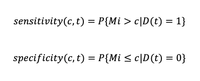The estimates for sensitivity (Se) and specificity (  at time t  are then calculated by combining the conditional KM estimator of the survival function, ), for the subset of Mi>c, and , the cumulative distribution function of the subset of Mi  (Cattaneo, 2017):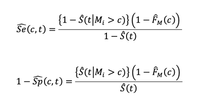In our macro, we used PROC LIFETEST to generate the KM estimator for .  is the conditional survival function for the subset of data where . We used the WHERE statement in PROC LIFETEST to restrict the data to those individuals whose predicted values meet the threshold criteria, c , and calculate the conditional survival function.  is the cumulative distribution function for the predicted values, . This is computed as the proportion of observations below the threshold c . We nested the procedure within a do loop to estimate the survival and cumulative distribution functions across all possible values for the threshold c.

``````%macro Sen_Spe;
/*This macro uses the dataset orig_z1 that contains predicted values for each individual. Orig_z1 is obtained from PROC PHREG, previously run with the baseline statement:
baseline out=orig_z xbeta=betaz covariates=orig timelist=&Timepoint;
orig is a dataset of individuals for which you want the predicted values*/
%let i = 1;
/*Calculate Sensitivity and Specificity at each criterion c*/
%do %until(%sysevalf(&&c&i. >= &m,boolean) = 1);
/*Estimate Conditional Survival Function Shat_c = S(t|M>c) at time T*/
proc lifetest data=orig_z1 method=KM outsurv=shat_c noprint;
where betaz > &&c&i..;
time &time2event_2*&event(0);
run;
proc sort data=shat_c;
by &time2event_2;
run;
/*Specify Timepoint T*/
data _null_;
set shat_c end=eof;
where &time2event_2 <= &Timepoint and not missing(survival);
if eof then call symput('shat_c',put(survival, best20.));
run;
/*Empirical Distribution Defined as Obs Below C Divided by Total Obs*/
proc sql;
select count(*) into: n_c from orig_z1 where betaz <= &&c&i.;
quit;
data Out;
c = &&c&i.;
/*Sensitivity*/
boot_sens = (1 - &shat_c)*(1 - &n_c/&n)/(1 - &shat);
/*Specificity*/
boot_spec = 1 - (&shat_c*(1 - &n_c/&n))/(&shat);
run;
proc append data=out base=res;
run;
%let i = %eval(&i+1);
%end;
data Out;
c = &&c&i.;
boot_sens = 0;
boot_spec = 1;
run;
proc append data=out base=res;
run;
%mend;
``````

The %Sen_Spe macro generates a dataset with the sensitivity and specificity for all possible threshold values at time t. The area under the curve, AUC(t):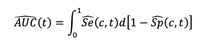can be estimated by applying the trapezoidal rule such that (Shiang, 2004):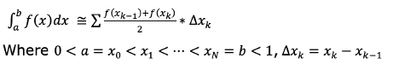``````/*Step 5 Calculate AUC(t), using data with sensitivity and specificity at each C.*/
proc sort data=res(rename=(Boot_sens = sens));
by descending boot_spec Sens;
run;
data area1;
set res end=last;
x = 1 - boot_spec;
xprev=lag(x);
yprev=lag(sens);
output;
if last then do;
xprev=x;
yprev=sens;
x=1;
sens=1;
output;
end;
run;
/*Apply Trapezoidal rule */
data _null_;
retain area 0;
set area1(firstobs=2) end=last;
area=area+(sens+yprev)*(x-xprev)/2;
if last then call symput('ROC_Boot',put(area, best20.));
run;
``````

# BOOTSTRAPPING

The bootstrap is a technique used to obtain estimates of statistics without making assumptions about the actual distribution of the data. It replicates the process of sample generation by drawing, with replacement, a sample of the observed data points of the same size as the original data. The newly generated data is called the bootstrap sample. In SAS, bootstrap samples can be generated by using PROC SURVERYSELECT with the replacement option (method=urs). Efron (Efron, 1993) introduced several bootstrap procedures for obtaining a nearly unbiased estimate of future model performance. In this paper, we apply the enhanced bootstrap method, which uses the difference between the AUC(t) estimated on the original data and the estimated AUC(t) from applying a model estimated in the bootstrap sample to the original data as the bias incurred from overfitting or “optimism”. This process is repeated and averaged to obtain the average optimism. The average optimism is subtracted from the AUC(t) calculated on the original sample to get the bias-corrected estimate of the AUC(t).

## METHOD

To implement internal validation of the AUC(t) , we bootstrap our sample B times. Then we estimate the AUC(t)b for each bootstrapped sample using the following steps:

• Using the original dataset, estimate the time-dependent sensitivity (Se0) and specificity (Sp0 ) from the Cox Proportional Hazards (Cox PH) model and calculate AUC(t)0.
• Select B independent bootstrap samples, by sampling the original data with replacement B times using PROC SURVEYSELECT. For each b = 1 ... B bootstrap sample:
1. Fit a Cox PH model and apply the parameter estimates to the original data to estimate Mbi and the range of threshold Cb.
2. Calculate the time-dependent sensitivity (SeBootb) and specificity (SpBootb) for each Cb.
3. Estimate AUC(t)b from the sensitivity (SeBootb) and specificity (SpBootb).

The following code generates N bootstrap samples using PROC SURVEYSELECT, fits the Cox PH model on the bootstrap sample (boot) and applies the parameter estimates to original sample to obtain Mbi (orig_z).

``````/*Bootstrap orginal sample*/
proc surveyselect data=&DT NOPRINT seed=&seed outhits
out=Boot
method=urs          /* resample with replacement */
samprate=1          /* each bootstrap sample has N observations */
reps=&Nboot; 	    /* generate Nboot bootstrap resamples */
run;
/*Cox model on Boot&n data*/
proc phreg data=Boot noprint;
by Replicate;
class &cls_var/ param=reference;
/*Apply parameter on original data*/
baseline out=orig_z xbeta=betaz covariates=orig timelist=&Timepoint;
run;
``````
• Estimate AUC(t)op optimism by the sample average of the B replications

##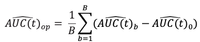• Final biased-correlated AUC(t) equals to

#THE %TIMEAUCBOOT MACRO

The %TIMEAUCBOOT macro parameters are shown in Table 1.

 DATA= (Required) SAS data set name. TIMEPOINT= (Required) Specific time point for AUC. EVENT= (Required) The outcome of interest. This has to be coded as 0 or 1, where 0 means censored and 1 means having the outcome of interest. TIME2EVENT= (Required) Time to the event (or censoring). ADJ_VAR= All covariates adjusted for in the model. CLS_VAR= (Required if categorical variable exist in ADJ_VAR) All categorical covariates from ADJ_VAR. The reference can be specified in this statement. NBOOT = The number of bootstrap samples. The default is 10. SEED= Random control seed. The default is 123. METHOD=KM Methods of estimating time-dependent AUC. Currently only the Kaplan-Meier method is available.

Table 1. Parameters for Macro %TIMEAUCBOOT

The Macro generates two figures, and one table. The Figure 1 displays the estimated bias within each bootstrap sample. The bias is calculated as the bootstrap AUC(t) minus the original AUC(t). The closer to zero, the less biased are the AUC(t) estimates from each bootstrap sample. The Figure 2 shows the distribution of the bootstrapped AUC(t). Ideally, we would like to see a distribution with a peak at a certain value rather than a flat distribution. Table 2 includes the time point which was specified to estimate the AUC(t). The pre-validation AUC(t) from the original sample and validated AUC(t).

The macro is available upon request from the author (Jialin Han) and is posted to GitHub website (https://github.com/hjlhanjialin/TIMEAUCBOOT). Once downloaded, use the %INCLUDE statement to specify the directory where the “TIMEAUCBOOT” macro is stored.

``%include "Directory/TIMEAUCBOOT.SAS";``

# EXAMPLE

This example uses a data from Transplant Readiness Assessment Clinic (TRAC), a transplant waitlist management strategy to evaluate a patient’s readiness for kidney transplant. (Cheng, 2018; Watford, 2020). The file contains data for 199 patients from 2017 to 2018. We are interested in assessing the predictive accuracy of a survival model that predicts the time to removal from the waitlist or death, adjusted for age group, gender and self-reported SF-36 physical functioning subscale scores. The data contains the following variables:

• ID: The system generated identification number
• REC_SF36_AVG: The average SF-36 scores
• REC_ISMALE: The gender indicator: 0=female and 1=male
• AGE_GRP: The age group variable. 1=age less than 45, 2=age between 45 and 70, and 3=above 70
• EVENT: The outcome 1=removal from waitlist or death, 0=censored prior to removal from waitlist or death
• FOLLOW_TIME: The time to the first event (censoring or outcome of interest).

The following statements create the TRAC dataset:

``````Data TRAC;
format age_grp agegr.;
Input ID REC_SF36_AVG REC_ISMALE AGE_GRP FOLLOW_TIME EVENT;
datalines;
1	50	0	2	259	1
2	75	1	2	40	1
3	90	1	2	185	0
4	85	1	1	620	0
5	80	1	2	625	0
6	75	0	2	480	0
7	80	0	2	314	0
8	20	0	2	730	0
9	90	0	2	415	0
10	95	1	2	385	0
11	40	0	2	532	0
12	60	0	2	101	0
13	25	0	2	7	1
14	85	0	2	511	0
15	80	0	1	183	0

... more lines ...

198	80	0	1	98	0
199	95	1	1	397	0
;
*The following code is used to run the macro to validate the time-dependent AUC.
%TIMEAUCBOOT(
DT		        =	TRAC,
TIMEPOINT 	= 	365,
CLS_VAR 	= 	AGE_GRP REC_ISMALE(REF = “1”),
TIME2EVENT 	= 	FOLLOW_TIME,
EVENT 	        = 	EVENT,
NBOOT 	        = 	100,
METHOD 	= 	KM,
SEED 		= 	123
);
``````

As shown in Figure 1, most of the values are negative suggesting that the original model performed better than the bootstrapped model, i.e., there was overfitting present. The distribution of the bootstrapped AUC(t) is shown in Figure 2 with values ranging between 0.75-0.80 with a few extreme values below 0.70. This suggests that the model performance is fairly stable.

In Table 2, the first two columns show the method (KM) and the timepoint (t=365 days) specified in the macro as the follow-up time for estimating the AUC(t). The pre-validation AUC(t) from the original sample equals to 0.79, the average bias or optimism equals to -0.02 and the final biased-corrected AUC(t) equals to 0.77. These results demonstrate that after internal validation, the final bias corrected AUC(t) is 0.77, which is slightly smaller than the original estimate but suggesting good performance.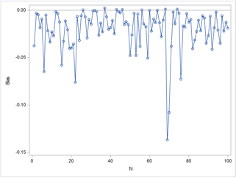Figure 1. Bias for Each Bootstrap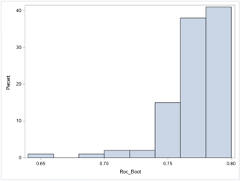Figure 2. Distribution of Bootstrap AUC(t)

 Method Time point AUC originalsample Over-optimism Bias-correctedAUC KM 365 0.79190 -0.020478 0.77142

Table 2. Final Output

# CONCLUSION

With the increased popularity of developing prediction models for survival outcomes it is important to have readily available tools to evaluate the performance of these models. The %TIMEAUCBOOT macro provides a useful and convenient tool for internal validation of the time-dependent AUC by using the bootstrap. Not only does it produce an estimate for the bias-corrected  it also displays the distribution of bias within each bootstrap sample. By using this tool researchers can evaluate the stability of the final model.

# REFERENCES

Heagerty, P. J., Lumley, T., and Pepe, M. S. (2000). “Time-Dependent ROC Curves for Censored Survival Data and a Diagnostic Marker.” Biometrics 56:337–344.

Uno, H., Cai, T., Tian, L., and Wei, L. J. (2007). “Evaluating Prediction Rules for t-Year Survivors with Censored Regression Models.” Journal of the American Statistical Association 102:527–537.

Steyerberg, EW., Harrell, FE., Borsboom, G., Eijkemans, M.J.C., Vergouwe, Y., Habbema, J.D.K. (2001) “Internal validation of predictive models: Efficiency of some procedures for logistic regression analysis” Journal of Clinical Epidemiology, Volume 54, Issue 8, Pages 774-781.

Guo, C., So, Y., and Jang, W. (2017). “Evaluating Predictive Accuracy of Survival Models with PROC PHREG” In SAS Conference Proceeding: SAS Global Forum 2017, Orlando, Florida: SAS Institute Inc.

Cattaneo, M., Malighetti, P., Spinelli, D. (2017) “Estimating Receiver Operative Characteristic Curves for Time-dependent Outcomes: The Stroccurve Package” The Stata Journal: 17(4):1015-1023.

Shiang, K. (2004) “The SAS® Calculations of Areas Under the Curve (AUC) for Multiple Metabolic Readings”, In SAS Conference Proceeding: Western Users of SAS Software 2004, Pasadena: SAS Institute Inc.

Efron, B. and Tibshirani, R. 1993. An Introduction to the Bootstrap. New York: Chapman and Hall.

Cheng, XS., Busque, S., Lee, J., Discipulo, K., Hartley, C., Tulu, Z., Scandling, JD., Tan, JC. (2018) “A new approach to kidney wait-list management in the kidney allocation system era: Pilot implementation and evaluation.” Clin Transplant. 2018 Nov;32(11):e13406.

Watford, DJ.Cheng, XS.Han, J.Stedman, MR.Chertow, GM.Tan, JC. (2021) “Toward telemedicine‐compatible physical functioning assessments in kidney transplant candidates.” Clin Transplant. 202135:e14173.

# ACKNOWLEDGMENTS

We would like to thank Dr. Jane Tan, Dr. Daniel Watford and Dr. Xingxing Cheng for use of the TRAC data which provided motivation for developing this macro. The John M. Sobrato Gift fund provided funding to support this project.

# CONTACT INFORMATION

Your comments and questions are valued and encouraged. Contact the author at:

Jialin Han

Stanford University

Colin-Jialin.han@stanford.edu

https://med.stanford.edu/profiles/jialin-han

Version history
Last update:
‎12-10-2021 02:14 PM
Updated by:Contributors
Article Labels
Article Tags